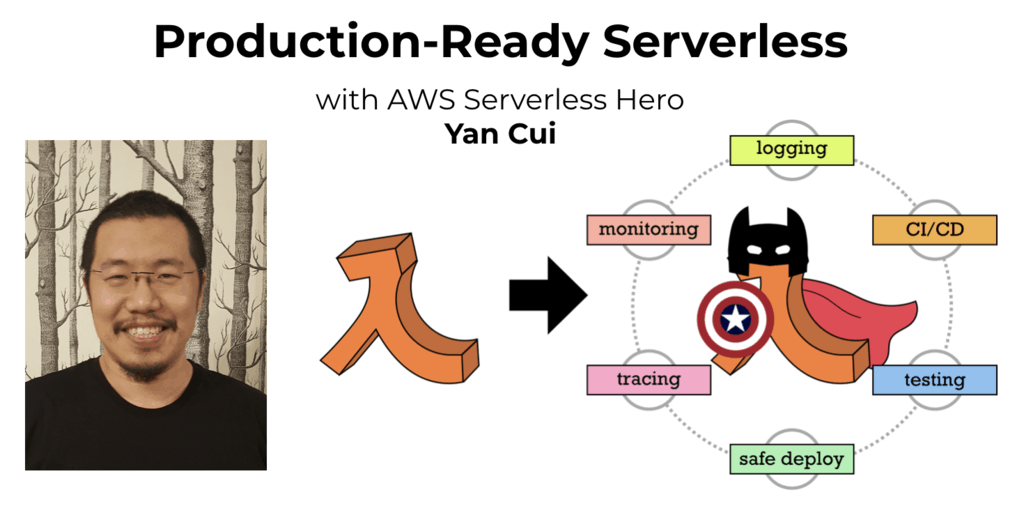# Project Euler – Problem 27 Solution

Check out my new course Learn you some Lambda best practice for great good! and learn the best practices for performance, cost, security, resilience, observability and scalability.

#### Problem

Euler published the remarkable quadratic formula:

n² + n + 41

It turns out that the formula will produce 40 primes for the consecutive values n = 0 to 39. However, when n = 40, 402 + 40 + 41 = 40(40 + 1) + 41 is divisible by 41, and certainly when n = 41, 41² + 41 + 41 is clearly divisible by 41.

Using computers, the incredible formula  n² – 79n + 1601 was discovered, which produces 80 primes for the consecutive values n = 0 to 79. The product of the coefficients, -79 and 1601, is -126479.

n² + an + b, where |a| < 1000 and |b| < 1000

where |n| is the modulus/absolute value of n

e.g. |11| = 11 and |-4| = 4

Find the product of the coefficients, a and b, for the quadratic expression that produces the maximum number of primes for consecutive values of n, starting with n = 0.

#### Solution

```let hasDivisor(n) =
let upperBound = int64(sqrt(double(n)))
[2L..upperBound] |> Seq.exists (fun x -> n % x = 0L)

// need to consider negative values
let isPrime(n) = if n <= 1L then false else not(hasDivisor(n))

let F (n:int64) (a:int64) (b:int64) = (n*n) + (a*n) + b

// function to return the number of consecutive primes the coefficients generate
let primeCount a b =
Seq.unfold (fun state -> Some(state, state + 1L)) 0L
|> Seq.takeWhile (fun n -> isPrime (F n a b))
|> Seq.length

let aList, bList = [-999L..999L], [2L..999L] |> List.filter isPrime

let (a, b, _) =
aList
|> List.collect (fun a ->
bList
|> List.filter (fun b -> a + b >= 1L)
|> List.map (fun b -> (a, b, primeCount a b)))
|> List.maxBy (fun (_, _, count) -> count)
a * b
```

Whilst I started off using a brute force approach (which took quite a while to run) I realised that most of the combinations of a and b don’t generate any primes at all. In order to reduce the number of combinations you need to check, consider the cases when n = 0 and n = 1:

n = 0: expression evaluates to b, in order for this to generate a prime b must be >= 2.

n = 1: expression evaluates to 1 + a + b, in order for this to generate a prime 1 + a + b must be >= 2, therefore a + b >= 1.Hi, I’m Yan. I’m an AWS Serverless Hero and the author of Production-Ready Serverless.

I specialise in rapidly transitioning teams to serverless and building production-ready services on AWS.

Are you struggling with serverless or need guidance on best practices? Do you want someone to review your architecture and help you avoid costly mistakes down the line? Whatever the case, I’m here to help.Check out my new course, Learn you some Lambda best practice for great good! In this course, you will learn best practices for working with AWS Lambda in terms of performance, cost, security, scalability, resilience and observability. Enrol now and enjoy a special preorder price of £9.99 (~\$13).Are you working with Serverless and looking for expert training to level-up your skills? Or are you looking for a solid foundation to start from? Look no further, register for my Production-Ready Serverless workshop to learn how to build production-grade Serverless applications!